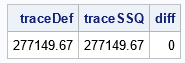Let X be any rectangular matrix. What is the trace of the crossproducts matrix, X'*X? Interestingly, you do not need to form the crossproducts matrix to compute the answer! It turns out that tr(X'*X) equals the sum of the squared elements of X.

Theorem: For any matrix, X, the trace of the crossproducts matrix is the sum of the squared elements: $\mathrm{tr}(X'X) = \sum_i \sum_j X_{ij}^2$.

Proof: By definition of matrix multiplication, the i_th diagonal element of $X'X$ is $(X'X)_{ii} = \sum_j (X')_{ij} X_{ji} = \sum_j X_{ji} X_{ji} = \sum_j X_{ji}^2$. Therefore, the trace of $X'X$ is $\mathrm{tr}(X'X) = \sum_i (X'X)_{ii} = \sum_i \sum_j X_{ji}^2$.

You can use the TRACE function and the SSQ function in the SAS IML matrix language to verify that the formula holds for the following example:

proc iml; /* read in any numeric matrix, X */ use sashelp.class; read all var {age weight height} into X; close;   traceDef = trace(X*X); /* slow: explicitly forms X*X */ traceSSQ = ssq(X); /* fast: does not forms X*X */ diff = max(abs(traceDef - traceSSQ));   reset fuzz=1E-10; /* print tiny numbers as 0 */ print traceDef traceSSQ diff;`The program shows that you can compute the trace of a crossproducts matrix directly from X without ever forming the crossproducts matrix. Notice that the traditional formation of X'*X requires O(n2m) when X is an n x m matrix. In contrast, the sum of the squared elements is O(nm).

Tags
Share